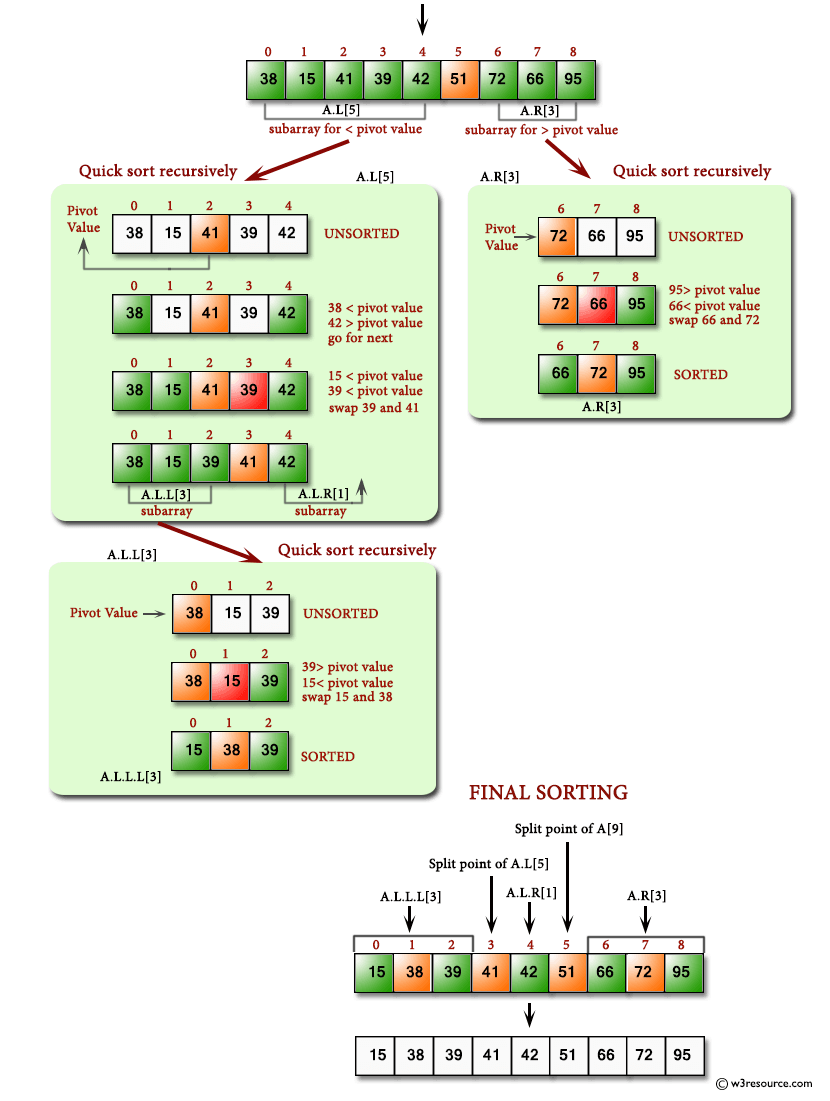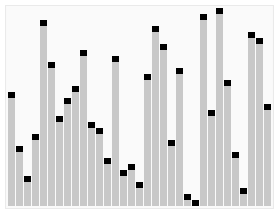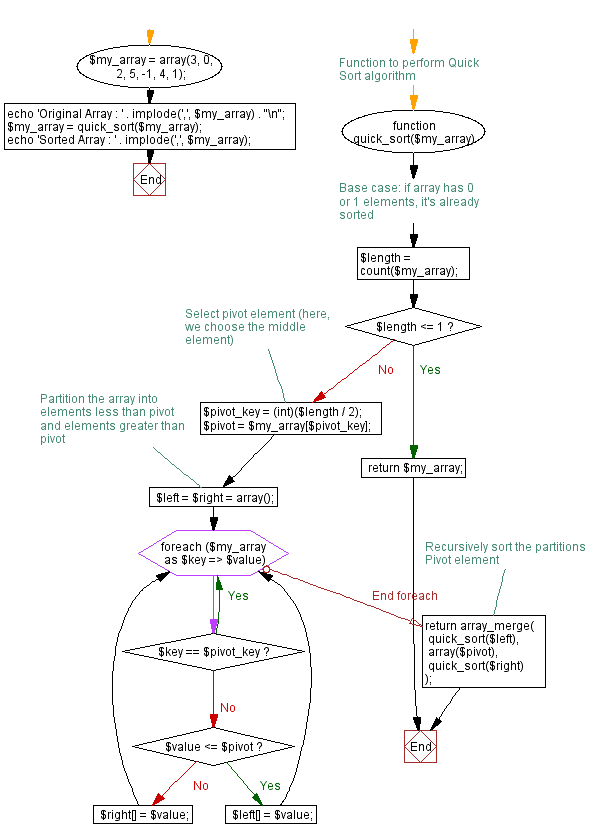﻿ PHP: Sort a list of elements using Quick sort - w3resource

# PHP Searching and Sorting Algorithm: Quick sort

## PHP Searching and Sorting Algorithm: Exercise-1 with Solution

Write a PHP program to sort a list of elements using Quick sort.

Quick sort is a comparison sort, meaning that it can sort items of any type for which a "less-than" relation (formally, a total order) is defined.

Pictorial presentation - Quick Sort algorithm :Animated visualization of the quicksort algorithm. The horizontal lines are pivot values.Animation credits : RolandH

Sample Solution:

PHP Code:

``````<?php
function quick_sort(\$my_array)
{
\$loe = \$gt = array();
if(count(\$my_array) < 2)
{
return \$my_array;
}
\$pivot_key = key(\$my_array);
\$pivot = array_shift(\$my_array);
foreach(\$my_array as \$val)
{
if(\$val <= \$pivot)
{
\$loe[] = \$val;
}elseif (\$val > \$pivot)
{
\$gt[] = \$val;
}
}
return array_merge(quick_sort(\$loe),array(\$pivot_key=>\$pivot),quick_sort(\$gt));
}

\$my_array = array(3, 0, 2, 5, -1, 4, 1);
echo 'Original Array : '.implode(',',\$my_array).'\n';
\$my_array = quick_sort(\$my_array);
echo 'Sorted Array : '.implode(',',\$my_array);
?>
```
```

Sample Output:

```Original Array : 3,0,2,5,-1,4,1
Sorted Array : -1,0,1,2,3,4,5
```

Flowchart :PHP Code Editor:

Have another way to solve this solution? Contribute your code (and comments) through Disqus.

What is the difficulty level of this exercise?

Test your Programming skills with w3resource's quiz.

﻿